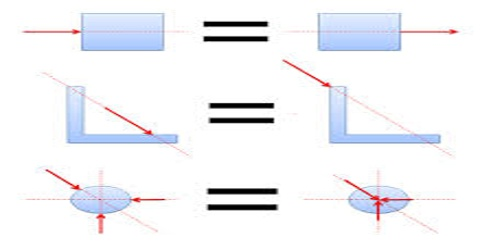# Independent Principle of Forces

Independent principle of forces:

According to Newton’s second law of motion rate of change of momentum, a body will be active along the direction of the force. So, the momentum that exists along the direction of the force will be changed with time only. Even in case of many forces effect of one force on the body will not be influenced by other forces. The characteristic or feature of the forces on a body is called the independent principle of forces or physical non-independence. Physical independence defines as vertical motion doesn’t affect by the movement of horizontal motion that phenomenon is known as the principle of physical independence of forces.

The reaction originated by any independent force towards the force or forces of interaction which originated the mentioned independent force is null. The independent action or actions originated by the interaction have limits that depend on the minimum and maximum speed reached in the interaction. The motion of a projectile is a two-dimensional motion. So, it can be discussed in two parts. Horizontal motion and vertical motion. These two motions take place independently of each other. This is called the principle of physical independence of motions. Here,

• The velocity of the particle can be resolved into two mutually perpendicular components. Horizontal component and a vertical component.
• The horizontal component remains unchanged throughout the flight. The force of gravity continuously affects the vertical component.
• The horizontal motion is a uniform motion and the vertical motion is a uniformly accelerated or retarded motion.

What we have learned from Newton’s second law of motion are:

(i) Acceleration of a body is proportional to the applied force on the body.

(ii) When the force does not act the body does not have acceleration retardation.

(iii) The direction of force is the direction of acceleration.

(iv) When a force acts on a body it mines with acceleration.